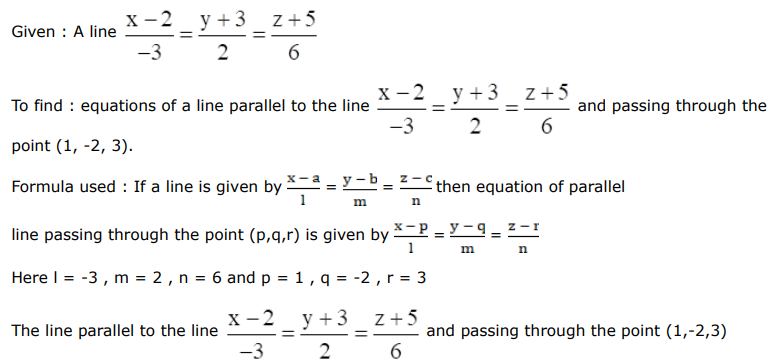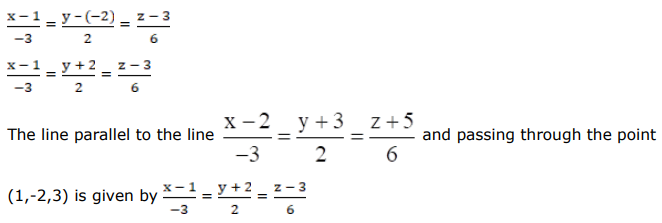# Write the equations of a line parallel to the lineQuestion:

Write the equations of a line parallel to the line $\frac{x-2}{-3}=\frac{y+3}{2}=\frac{z+5}{6}$ and passing through the point $(1,-2,3)$.

Solution:is given by